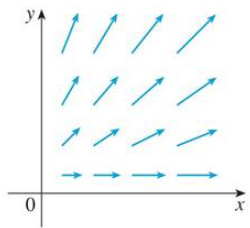Chapter 16.5, Problem 10E

Chapter
Section
Textbook Problem

The vector field F is shown in the xy-plane and looks the same in all other horizontal planes. (In other words, F is independent of z and its z-component is 0.)(a) Is div F positive, negative, or zero? Explain.(b) Determine whether curl F = 0. If not, in which direction does curl F point?10.(a)

To determine

To find and explain: The div F.

Explanation

Formula used:

Consider the standard equation of a divergence of vector field for F=Pi+Qj+Rk

divF=Px+Qy+Rz (1)

As F is independent of z-component (R=0) is Rx=Ry=Rz=0 .

From Figure, P and Q doesn’t vary in the z-direction, so Pz=Qz=0 and as the x increases, the each vector of F in x-component increases while the y-component remains constant, so Px>0 and Qx=0 .

Similarly, as the y increases, the each vector of F in y-component increases while the x-component remains constant, so Qy>0 and Py=0

(b)

To determine

Whether curl F=0 or not.

Still sussing out bartleby?

Check out a sample textbook solution.

See a sample solution

The Solution to Your Study Problems

Bartleby provides explanations to thousands of textbook problems written by our experts, many with advanced degrees!

Get Started

Convert the expressions in Exercises 6584 to power form. 4x23+x3/2623x2

Finite Mathematics and Applied Calculus (MindTap Course List)

In Exercises 43-46, find f(a + h) f(a) for each function. Simplify yoiir answer. 44. f(x)=12x+3

Applied Calculus for the Managerial, Life, and Social Sciences: A Brief Approach

For f(x) = 10x2, f′(3) = _____. 10 20 30 60

Study Guide for Stewart's Single Variable Calculus: Early Transcendentals, 8th

True or False: The function f is continuous at (0, 0), where

Study Guide for Stewart's Multivariable Calculus, 8th

Prove Equation (4), P(ABC)=P(A)P(BA)P(CAB)

Finite Mathematics for the Managerial, Life, and Social Sciences